• These are standard colors for our Exterior Window Shutters.
• We have the ability to match your existing shutter color for an upcharge.  Please provide paint Mfr. name and Color Code.  i.e.  Sherwin-Williams lists their colors like this:  SW 6747.
• Please Note:  Actual colors may vary slightly from those shown on the screen simply due to screen resolution, lighting, surface, or application background.B L A C KC H A R L E S T O N   G R E E NR O O K W O O D   S H U T T E R   G R E E N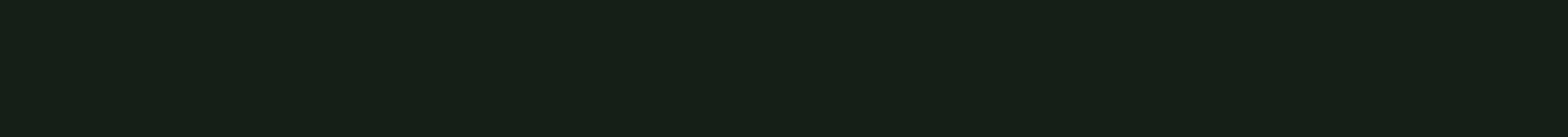R O Y C R A F T   B O T T L E   G R E E N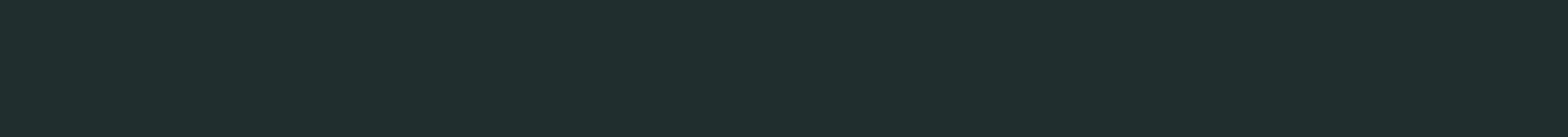E V E R G R E E N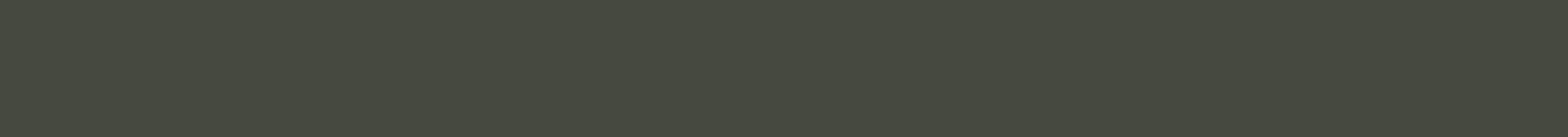R O O K W O O D   D A R K   G R E E N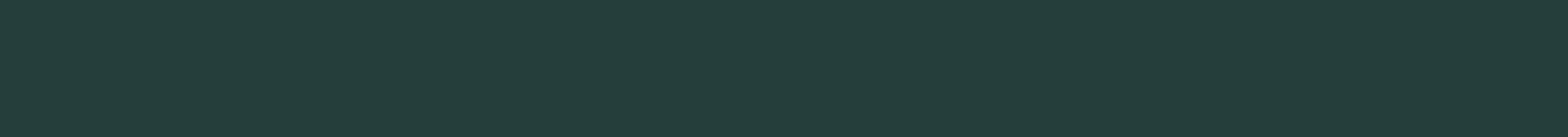F O R E S T   G R E E N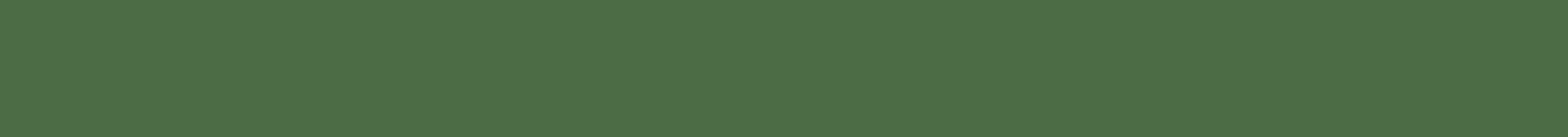P I N EM I D N I G H T   S K YD E E P   S E A   B L U ET E M P E S T   B L U EH A M I L T O N   B L U E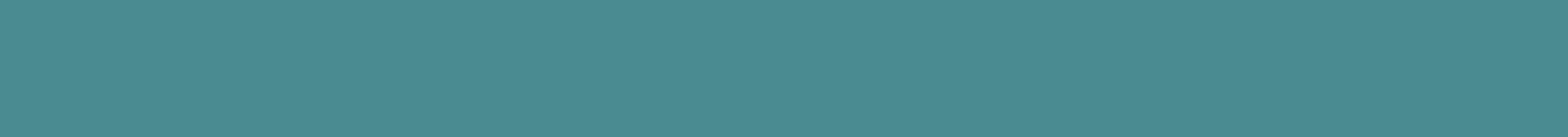H A R B O R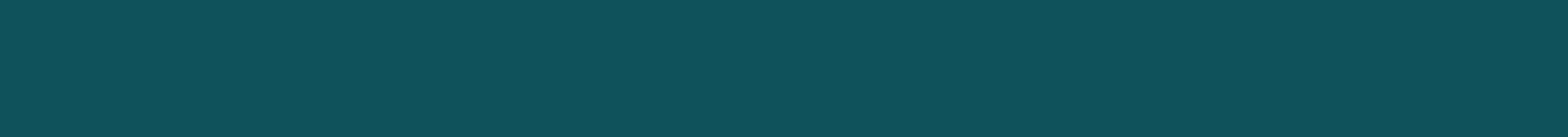C A S C A D EP L U M   C R E E KB O R D E A U XR O Y C R A F T   C O P P E R   R E DB O A R D   A N D   B A T T E N   R E D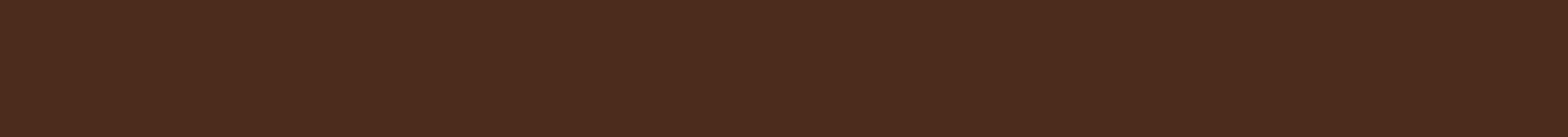F E D E R A L   B R O W N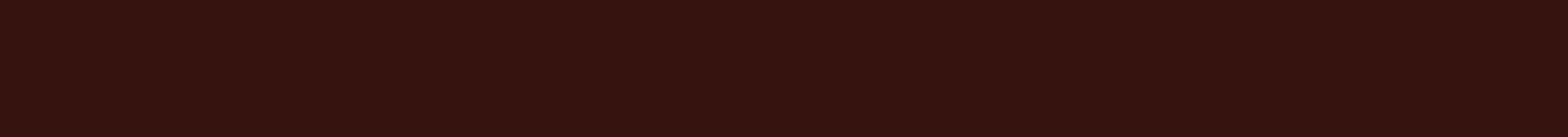P O L I S H E D   M A H O G A N Y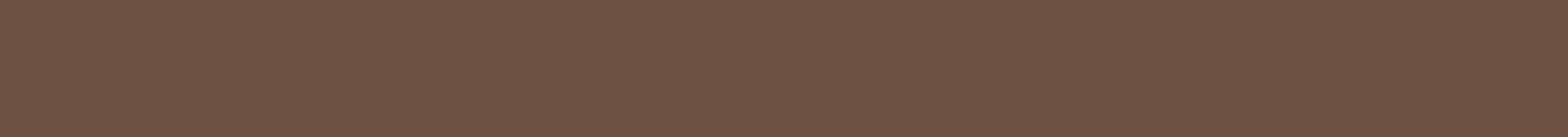R O O K W O O D   M E D I U M   B R O W NW A L N U T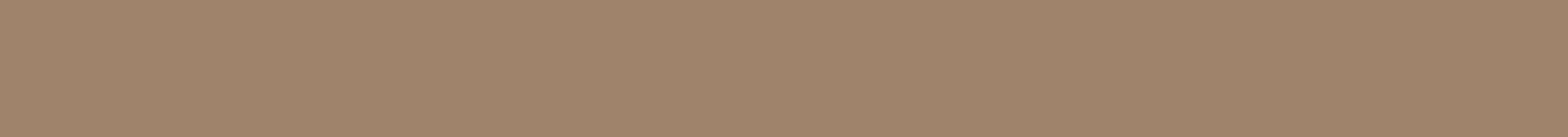C R A F T S M A N   B R O W N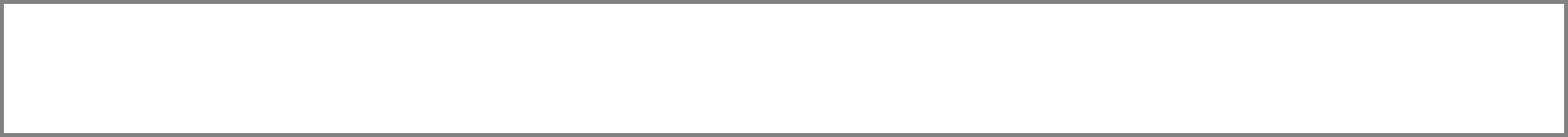W H I T ER O Y C R A F T   P E W T E R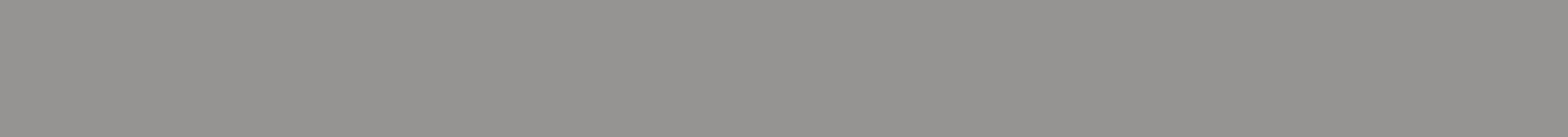P E W T E R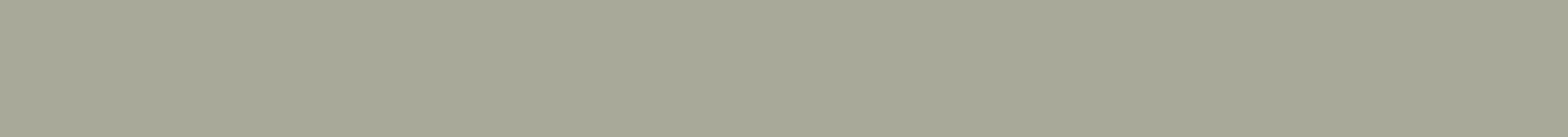R O Y C R A F T   M I S T   G R A Y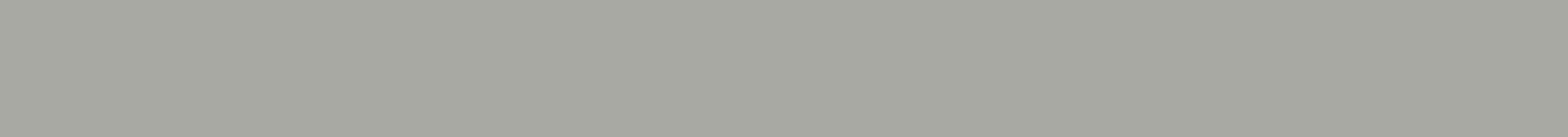C H E L S E A   G R A Y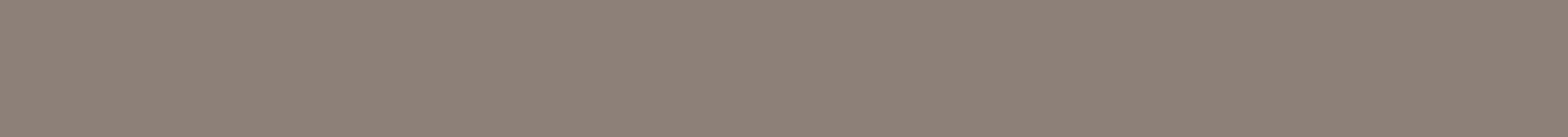H A M M E R E D   S I L V E R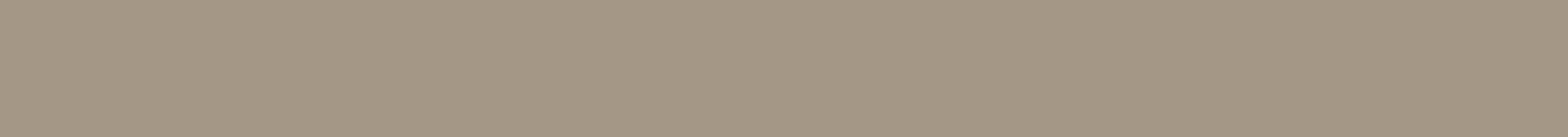S A N D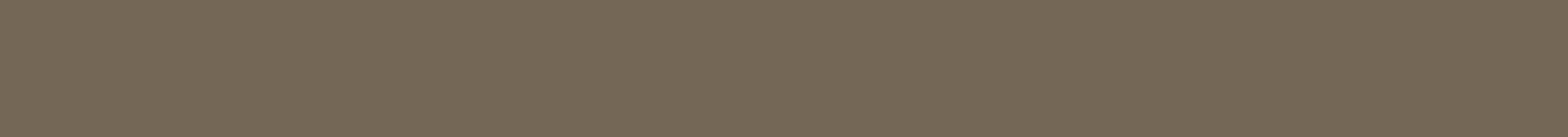W E A T H E R E D   S H I N G L E